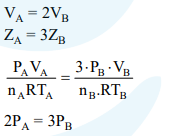# The volume of gas A is twice than that of gas B.

Question:

The volume of gas A is twice than that of gas B. The compressibility factor of gas A is thrice than that of gas B at same temperature. The pressures of the gases for equal number of moles are :

1. $2 \mathrm{P}_{\mathrm{A}}=3 \mathrm{P}_{\mathrm{B}}$

2. $\mathrm{P}_{\mathrm{A}}=3 \mathrm{P}_{\mathrm{B}}$

3. $\mathrm{P}_{\mathrm{A}}=2 \mathrm{P}_{\mathrm{B}}$

4. $3 \mathrm{P}_{\mathrm{A}}=2 \mathrm{P}_{\mathrm{B}}$

Correct Option: 1

Solution: